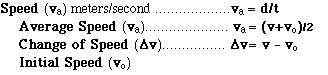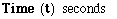# Hands-On-Physics

### GLOSSARYSpeed is simply the rate of position change, but for analysis and problem solving speed has several faces. The speed at an instant is can be approximated with an average speed measured over a short time interval. Initial speed is the speed of an object at the beginning of a measurement, an intial condition. The average speed is the ratio of distance covered to the time it takes, or the average of the final and intial speeds. The change in speed is the difference between final and inital speeds. The symbol v is used for speed because speed is the size of the velocity vector.

### Temperature (T)

You cannot measure heat directly, but you can detect its effect on a substance. Changes in heat can usually be detected as changes in temperature. When you add heat energy to a substance, it usually warms (temperature increases) ; when you remove heat energy it usually cools(temperature decreases).
In the SI system, temperature is measured in degrees Celsius . In the U.S. the old Fahrenheit scale is used.Time is one of the undefined qualities of physics. We all know what it is and agree to use a second as one unit of time.

A | B | C | D | E | F | G | H | I | J | K | L | M | N
O | P | Q | R | S | T | U | V | W | X | Y | Z
Top of Glossary